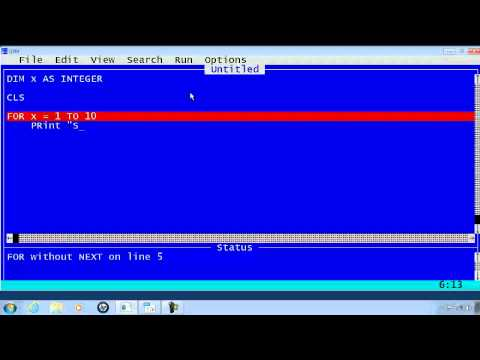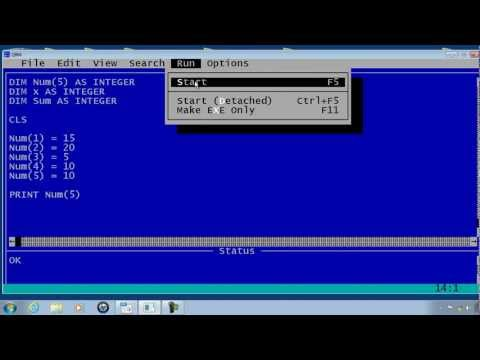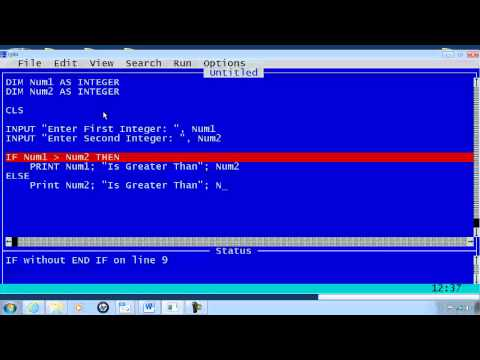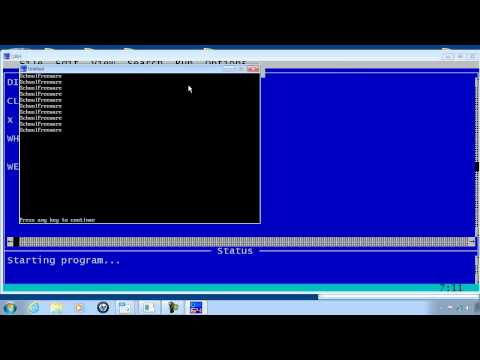## QBASIC Functions and Statements

Subject: Computer Science

#### Overview

Loop is a statement which executes one or more lines of code in certain number of times. Similarly, An array is a special type of variable with an unique name, size and type. This note provides an information about Qbasic Functions and statements.

#### Loop

Loop is a statement which executes one or more lines of code in certain number of times. The loop ends with the false condition. Every loop statement requires the following terms:

• Counter:It is a variable which controls the loop statement and provides the track to run the loop statement in certain number of times.
• Initial Value:The string value of loop statement is known as initial value.
• Sentient Value:This is the final value of loop statement.
• Step value:It is used to modify the value of counter by certain amount.

If...GOTO LOOP

This program use IF----GOTO to create a loop.

x= 10
start:
Print x
x= x+1 ( This adds 1 to x)
If x < 15 Then GOTO start
Output
10
11
12
13
14

For.....NEXT

It is the count controlled loop which knows how many times the loop is to be executed. The loop is executed with the loop control variable at initial value to final value and values in between.WHILE....WEND

It is a loop statement that executes a series of statements in a loop as long as the given statement is true.

#### ARRAY

An array is a special type of variable with an unique name, size and type. In another words, an array is a data structure that stores multiple values of similar type with a common name bu having different subscript. An array is defined by using DIM statement. There are two types of array:

• Single Dimensional Array: An array having series of similar type of data represented by a variable with a single subscript or index value known as single dimensional array. Example: DIM NUM (6), It defines a single dimensional array having named NUM having six sub-scripted variables.
• Multidimensional Array: An array having series of similar type of data represented by a variable with double subscripts is known as multidimensional array.

DIM

Syntax: DIM [ array name] [ value ]
Example:DIM kul (5)

#### The Branching Statement

The branching statement is decision making statement, depending upon the decision it can change the order of execution.

IF......THEN Statement

It helps the computer to check whether the relation is TRUE or FALSE.
Syntax: Line Number IF ( relational expression) THEN n where n is a line number or an instruction. If the expression is true then n will be executed otherwise statement following IF..THEN statement will be executed.

IF....THEN....ELSE

It is a decision making statement as it decides the path of the program.
Syntax: If (Condition) ...THEN (Line number or instruction) .....ELSE (Line number or instruction)

IF_ _ _ _ _ ELSEIF Statement

Syntax: IF ( Condition ) THENStatement-1ELSEIF (Condition) THENStatement-2ELSEIF (Conditon) THENStatement-ELSEStatement-4ENDIF

SELECT-CASE Statement

Syntax: SELECT CASE ( expression )
Statement-1
Case ( expression )
Statement-2
Case ( expression )
Statement-3
Case ELSE
Statement-4
END SELECT

#### Numeric Functions

Numeric functions are the type of library function which are used to process numeric or string data and always returns numeric value.

Some numeric functions are as follows:

• LEN FUNCTION: It is string processing function that return the number of characters in a string and it also returns the number of bytes required by a variable.
• ASC FUNCTION: It returns the ASCII (AMERICAN STANDARD CODE FOR INFORMATION INTERCHANGE) code of the first character of a string expression.
• VAL FUNCTION: It converts a string expression consisting of numeric digits into number and returns the number a value.
• ABS FUNCTION: It returns the absolute value of numeric expression or converts a negative number to a positive number.
• INT FUNCTION: It rounds and returns the largest integer less than or equal to a numeric expression.
• FIX FUNCTION: It returns the integer part of numeric expression.
• CINT FUNCTION: It converts a numeric expression to an integer by rounding the fractional part of the expression.
• SGN FUNCTION: It returns the sign of the number.
• SQR FUNCTION: It returns the square root of any positive number.
• SIN FUNCTION: It returns the sine of a specified angle.
• COS FUNCTION: It returns the cosine of a specified angle.
• TAN FUNCTION: It returns the tangent of a specified angle.
##### Things to remember
• Loop is a statement which executes one or more lines of code in certain number of times.
• An array is a special type of variable with an unique name, size and type. In another words, an array is a data structure that stores multiple values of similar type with a common name bu having different subscript.
• The Branching Statement
• The branching statement is decision making statement, depending upon the decision it can change the order of execution.
• Numeric functions are the type of library function which are used to process numeric or string data and always returns numeric value.
• It includes every relationship which established among the people.
• There can be more than one community in a society. Community smaller than society.
• It is a network of social relationships which cannot see or touched.
• common interests and common objectives are not necessary for society.
##### Videos for QBASIC Functions and Statements##### QBasic Tutorial 11 - For Loop - QB64##### QBasic Tutorial 15 - Arrays - QB64##### QBasic Tutorial 7 - IF Statements - QB64##### QBasic Tutorial 9 - While Loop - QB64
Using SUB Procedure:
DECLARE SUB SUM(A$) CLS INPUT"Enter any string";A$
CALL SUM(A$) END SUB SUM(A$)
FOR I = 1 to LEN(A$) B$= Ucase$(MID$(A$,I,1)) S=S+ASC(B$)
NEXT I
PRINT"Sum of ASCII value";S
END SUB
Declare Sub Kul ( )
CLS
Call kul ( )
END
Sub kul ( )
C=1
While C <= 10
Print C
C= C+1
Wend
End
DECLARE SUB Array( a( ) )
CLS
DIM a(5)
FOR I = 1 to 5
INPUT" Enter any number"; a(i)
NEXT I
CALL Array( a( ) )
END
SUB Array ( a( ) )
FOR I = 1 to 5
PRINT a(i)
NEXT I
END SUB
DECLARE FUNCTION Kins( a( ) )
CLS
DIM a(5)
FOR I = 1 to 5
INPUT"Enter the number"; a(i)
NEXT I
PRINT kins ( a ( ) )
FOR I = 1 to 5
SUM = SUM + a(i)
NEXT I
Kins= SUM
END FUNCTION
DECLARE SUB Even (n( ) )
CLS DIM n(5)
FOR I= 1 to 5
INPUT" Enter the number"; n(i)
NEXT I
CALL Even ( n ( ) )
END
SUB Even( n ( ) )
END
SUB Even (n ( ) )
FOR I = 1 to 5
R= n(i) mod 2 =0
SUM = SUM + n(i)
NEXT I
PRINT"Sum of even numbers is";SUM
END SUB

DECLARE SUB Array (n( ) )
CLS
DIM n(10 )
FOR I= 1 to 10
INPUT" Enter any number";n(i)
NEXT I
CALL Array( n ( ) )
END
SUB Array (n( ) )
END
SUB Array ( n ( ) )
FOR I = 1 to 10
IF n(i) > max THEN max= n(i)
NEXT I
PRINT" Maximum number";Max
END SUB
Using SUB Procedure:
DECLARE SUB Greatest(A,B,C)
CLS
INPUT"Enter the first number";A
INPUT"Enter the second number";B
INPUT"Enter the third number";C
CALL Greatest (A,B,C)
END
SUB Greatest(A,B,C)
IF A >B AND A > C THEN
PRINT"A is greatest"
ELSEIF B > A AND B > C THEN
PRINT"B is greatest"
ELSE
PRINT"C is greatest"
ENDIF
END SUB
DECLARE FUNCTION Smallest$( A,B,C) CLS INPUT"Enter the first number";A INPUT"Enter the second number";B INPUT"Enter the third number";C PRINT Smallest$ (A,B,C)
END
FUNCTION Smallest$( A,B,C) IF A < B AND A < C THEN Smallest$="A is smallest"
ELSEIF B < A AND B < C THEN
Smallest$="B is smallest" ELSE Smallest$= "C is smallest"
END
DECLARE SUB Per( P )
CLS
CALL Per ( P )
END
SUB Per ( P )
SELECT CASE P
CASE is < 30
PRINT"You are fail"
CASE 31 to 60
PRINT"You are third"
CASE 61 to 75
PRINT"You are second"
CASE 76 to 85
PRINT"You are first"
CASE ELSE
PRINT"You are distinction"
END SELECT
END SUB
Using FUNCTION Procedure:
DECLARE FUNCTION Age$(A) CLS INPUT"Enter your age";A PRINT Age$(A)
END
FUNCTION Age$(A) IF A >= 18 THEN Age$= "You can give vote"
ELSE
Age\$= "You can't give vote"
ENDIF
END FUNCTION